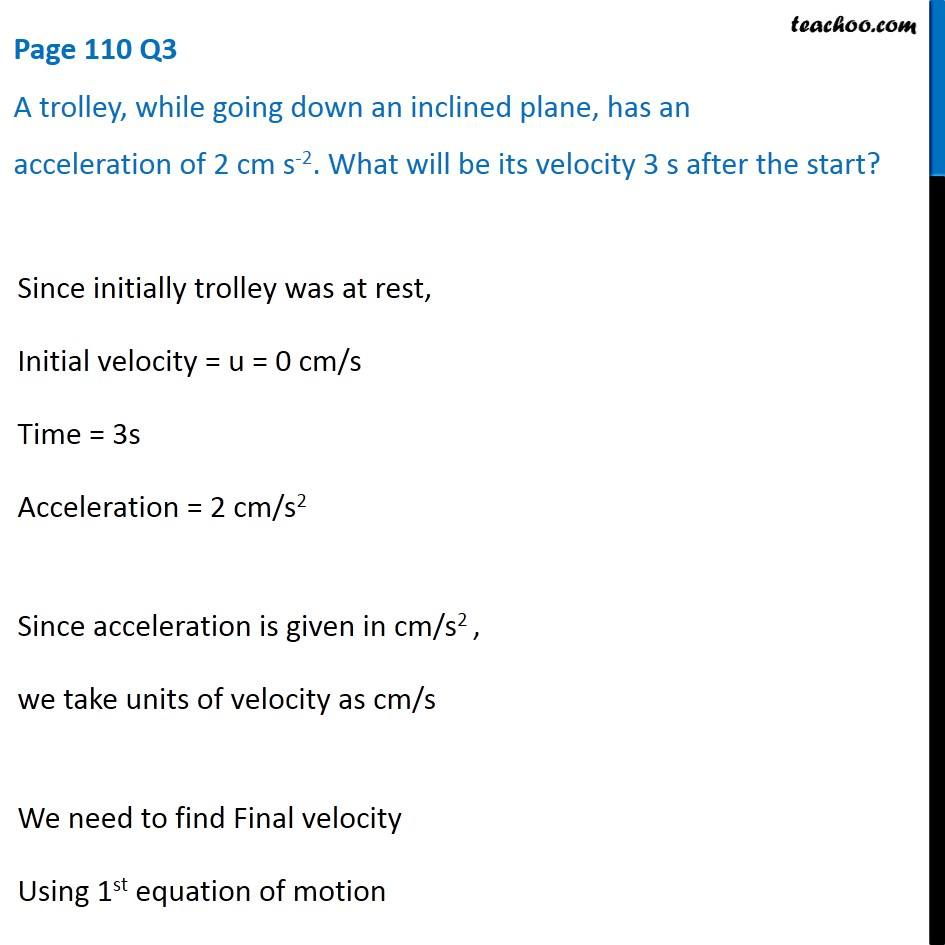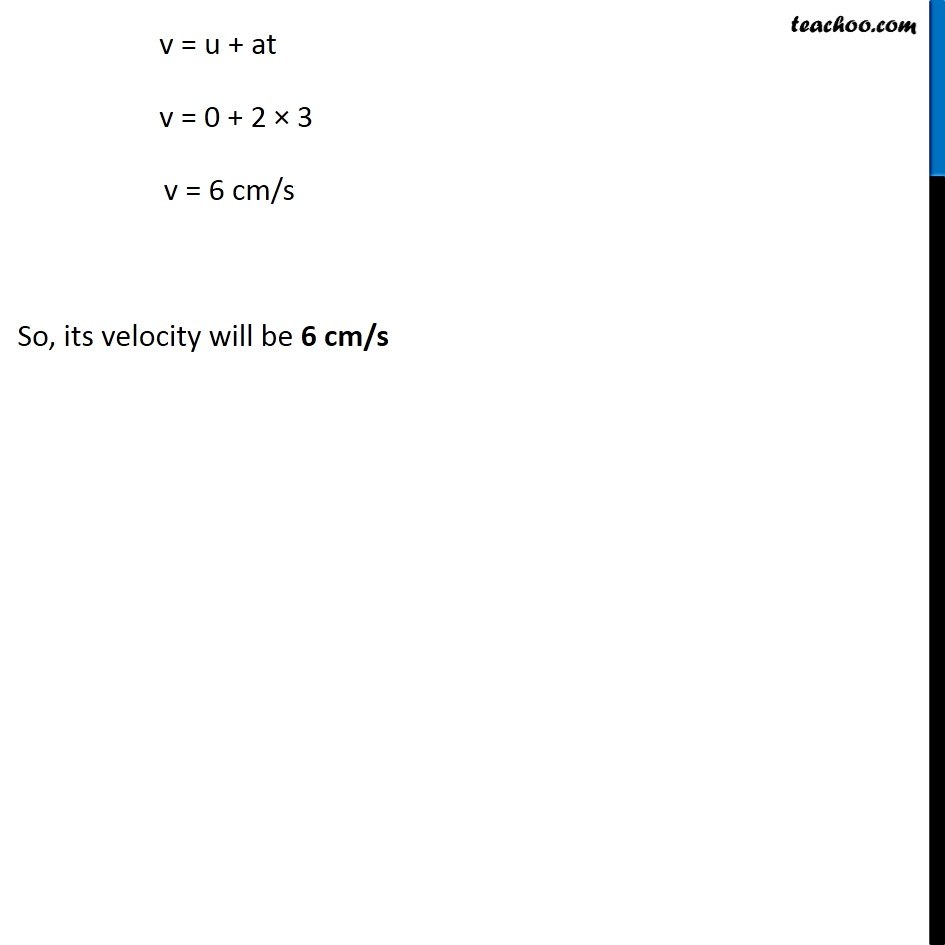Questions from Inside the chapter

Class 9
Chapter 8 Class 9 - MotionLearn in your speed, with individual attention - Teachoo Maths 1-on-1 Class

### Transcript

Page 110 Q3 A trolley, while going down an inclined plane, has an acceleration of 2 cm s-2. What will be its velocity 3 s after the start? Since initially trolley was at rest, Initial velocity = u = 0 cm/s Time = 3s Acceleration = 2 cm/s2 Since acceleration is given in cm/s2 , we take units of velocity as cm/s We need to find Final velocity Using 1st equation of motion v = u + at v = 0 + 2 × 3 v = 6 cm/s So, its velocity will be 6 cm/s